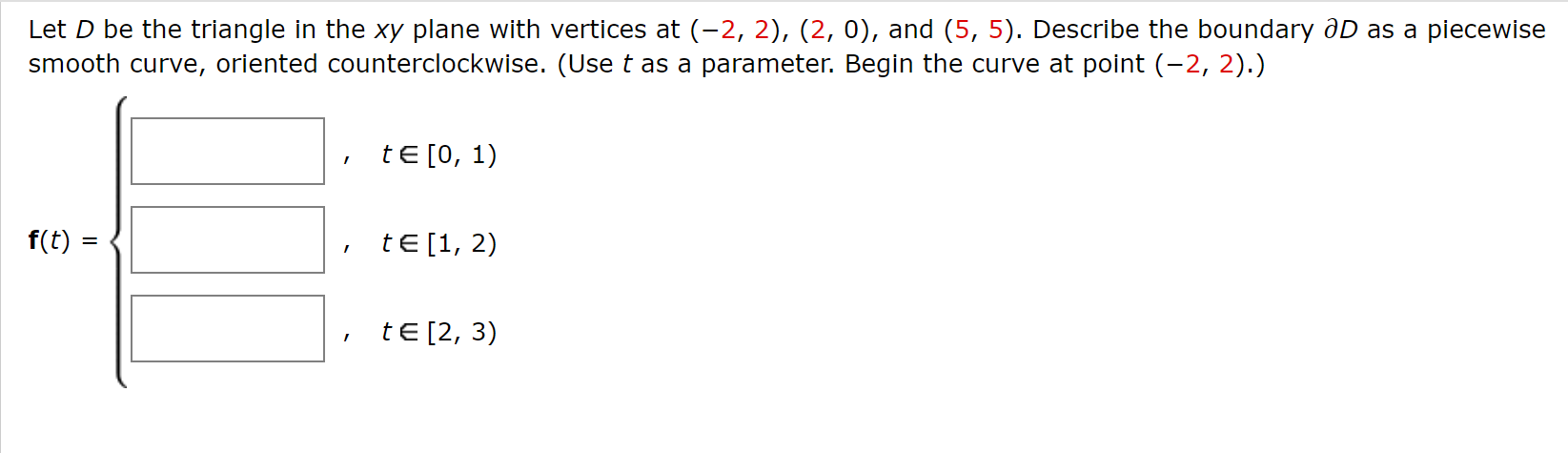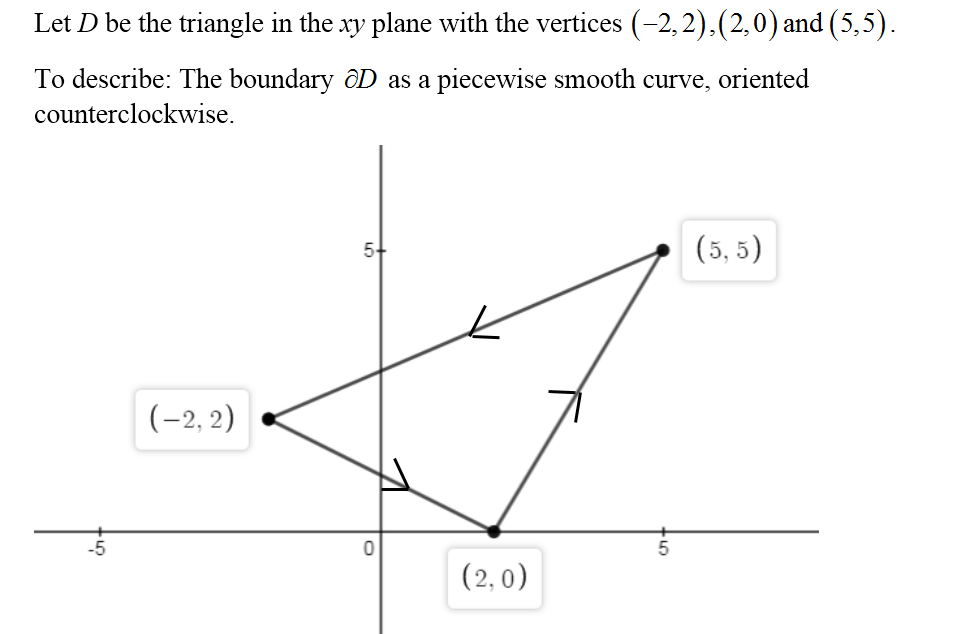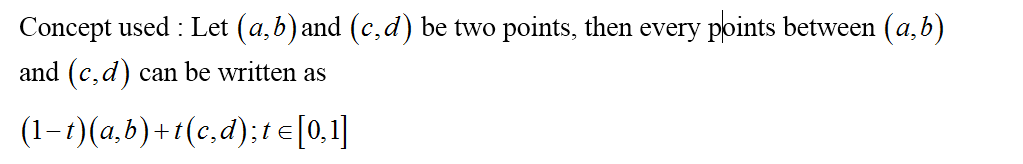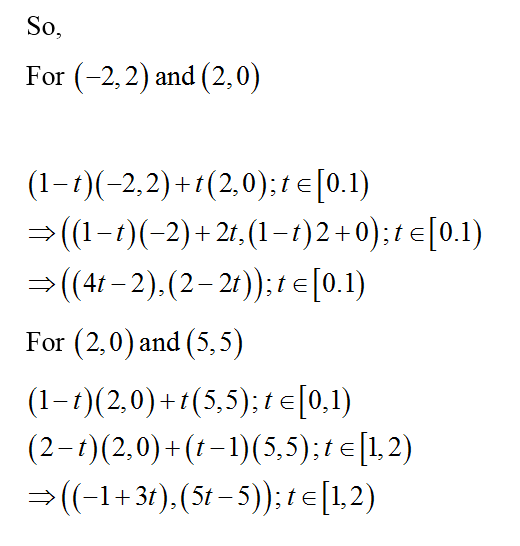# Let D be the triangle in the xy plane with vertices at (-2, 2), (2, 0), and (5, 5). Describe the boundary aD as a piecewisesmooth curve, oriented counterclockwise. (Use t as a parameter. Begin the curve at point (-2, 2).)tE [0, 1)f(t) :tE [1, 2)tE [2, 3)

Question
8 viewshelp_outlineImage TranscriptioncloseLet D be the triangle in the xy plane with vertices at (-2, 2), (2, 0), and (5, 5). Describe the boundary aD as a piecewise smooth curve, oriented counterclockwise. (Use t as a parameter. Begin the curve at point (-2, 2).) tE [0, 1) f(t) : tE [1, 2) tE [2, 3) fullscreen
check_circle

Step 1Step 2Step 3...

### Want to see the full answer?

See Solution

#### Want to see this answer and more?

Solutions are written by subject experts who are available 24/7. Questions are typically answered within 1 hour.*

See Solution
*Response times may vary by subject and question.
Tagged in